In this section you will learn about the properties of Quadrilaterals (2D Shapes).

In addition to the images below, this video below looks at the properties of quadrilaterals.

Quadrilaterals as their name suggests all shapes that have four sides. The most common quadrilaterals are listed below.

Square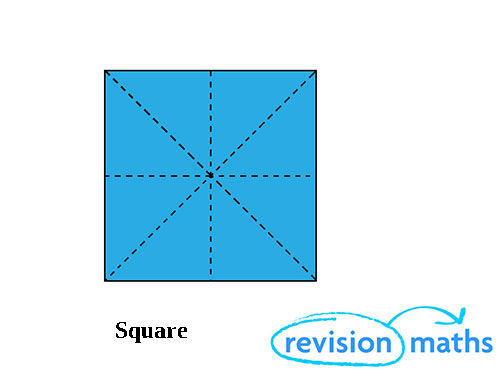Sides: Four equal sides, all four angles are equal (900). A square is therefore a regular quadrilateral.

Symmetry: Four lines, rotational order 4.

Rectangle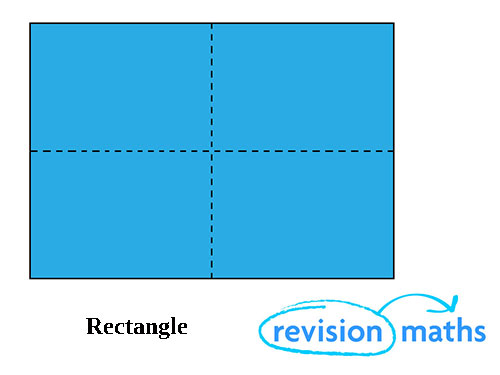Sides: Two pairs of opposite sides equal, all four angles are equal (900).

Symmetry: Two lines, rotational order 2.

Parallelogram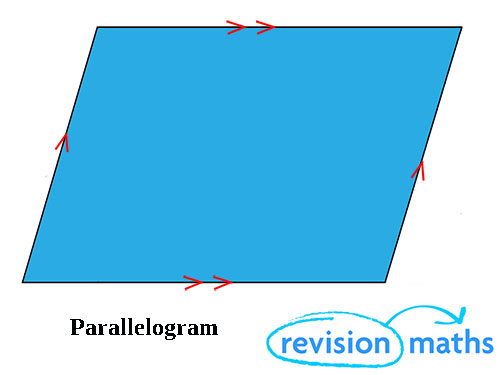Sides: Two pairs of opposite sides equal and parallel.

Symmetry: No lines, rotational order 2.

Rhombus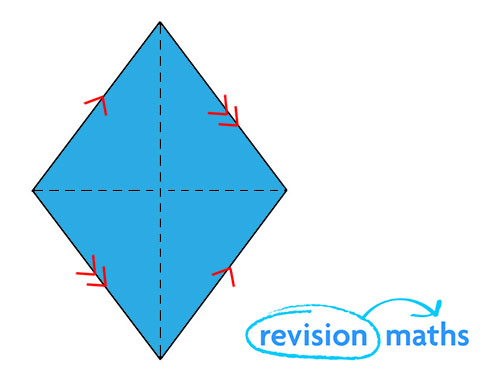Sides: Four sides equal, two pairs of opposite sides parallel.

Symmetry:  Two lines, rotational order 2.

Trapezium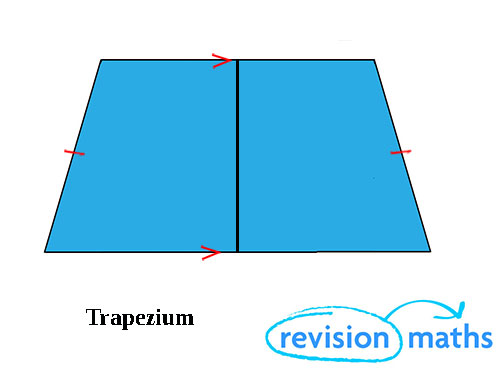Sides: One pair of parallel sides and can also be isosceles as in the example above.

Symmetry: One line in the example above, rotational order 1.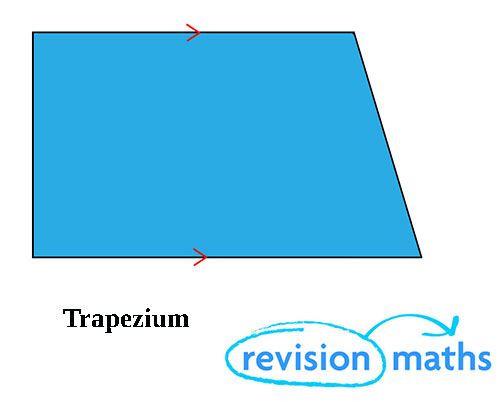Sides: One pair of parallel sides in the example above.

Symmetry: No lines in the example above, rotational order 1.

Kite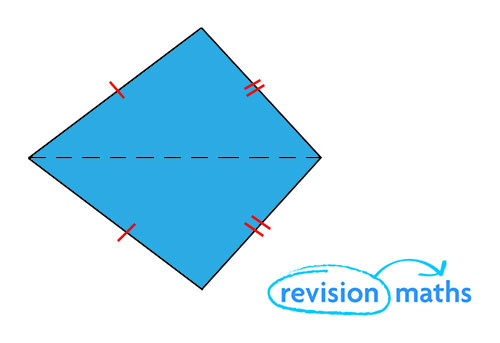Sides: Two pairs of adjacent sides equal.

Symmetry: One line, rotational order 1.